# Very Simple Amplifier with Phantom Power

## NEC 2SA992

The circuit in Figure 1 is suited for inductive sources such as a dynamic microphone, transformer or inductance sensor/pick up.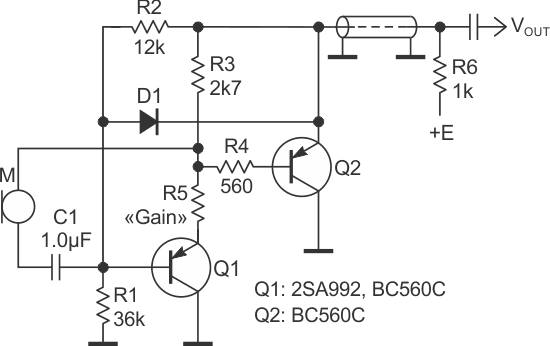Figure 1. A simple amplifier circuit suited for inductive sources such as a dynamic microphone, transformer or inductance sensor/pick up.

The circuit is versatile; its gain can be varied from 1 to about 130. it also has a wide power range (approximately 5 to 15 V). It uses no electrolytic capacitors, which improves reliability.

A low-impedance dynamic microphone can be connected to the input with or without any matching transformer. The circuit is powered through a two-wire output cable, i.e., the load resistor is separated from the circuit by a (screened) cable.

The maximum gain is about 130. This rather high value is provided by the common emitter input stage (low noise transistor Q1) with dynamic load R3, Q2.

While the maximum gain is achieved when R5 = 0 Ω, the gain can be reduced by choosing a non-zero value of resistor R5, which introduces a local feedback. Such local feedback also improves the circuit linearity.

For example, when R5 = 180 Ω, gain is about 60. The emitter follower (Q2) also provides a low output resistance for the circuit.

Like any other device connected to an inductance or long connecting cable, the circuit can be subject to overvoltages on its terminals. Diode D1 protects both input and output to avoid the related damage.

To calculate values of resistors we can begin with values of the power voltage E, collector current of Q1, and value of load resistor R6.

For example let E = 9 V and IC1 = 0.3 mA, then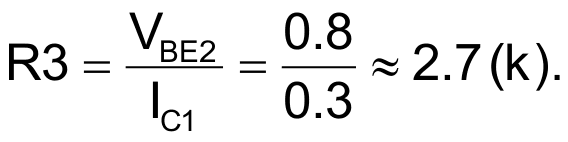To provide the needed output headroom we can choose the emitter voltage of Q2 as one-half of the power voltage: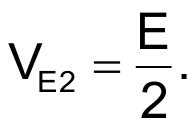Then we have a simple equation: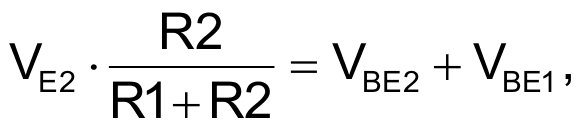from which the ratio R1/R2 can be obtained.

Since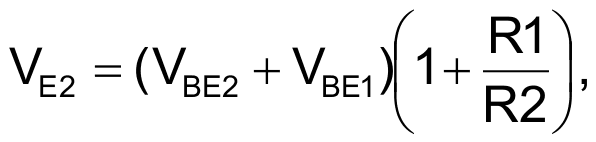and doesn’t depend on the value of E, the left-part of the circuit on DC represents a kind of Zener diode, which should be taken into account when you intend to raise the value of E.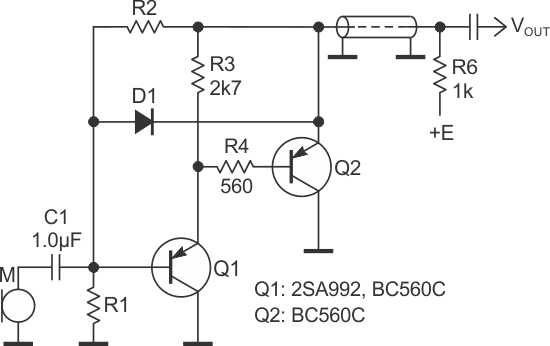Figure 2. The modified circuit to get a gain of about 1.

Figure 2 shows how the circuit can be modified to get the gain of about 1.

EDN